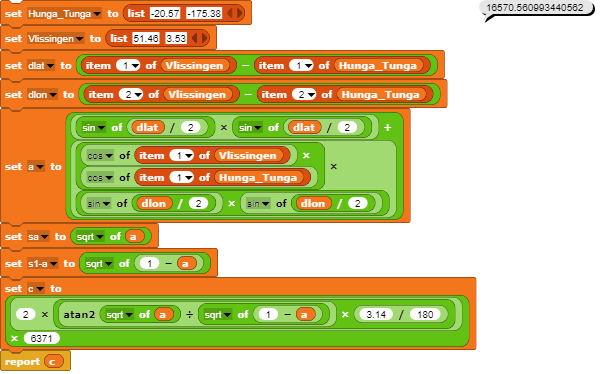# Haversine formula

I want to calculate the speed of the Hunga Tunga shockwave when it travelled the earth. I have the air pressure values from a local weather station for 15 and 16 January where the spikes of the Hunga Tunga eruption are clearly visible. My question: I need the distance from my weather station to the Hunga Tunga volcano without looking it up on the web. Tried to calculate the distance with the Haversine formula...but the atan2 block doesn't work for me.

I hope someone can help....thank you.
Here is the project so far:
https://snap.berkeley.edu/project?user=horowitz&project=Haversine%20formula

Hans

Is this a homework assignment? :~)

Because we measure angles clockwise from north rather than counterclockwise from east, we end up using atan2(x,y) rather than atan2(y,x). See if reversing the two inputs fixes it for you.

That didn't work, no it's not a homework assignment. I put the same code in python witch gives me 16673.39 km as distance. In Snap due the atan2 function I get 21434 km distance. Is the Snap atan2 function some special kind of function? in stead atan2(y,x)?

Process.prototype.reportBasicAtan2 = function (a, b) {
return degrees(Math.atan2(+a, +b));
};


If you don't know how to read this then it basically means that it uses the native JS atan2 function and isn't anything special

Converting the output to radians gives me 373.9.

Edit: Using a more accurate approximation of $$\pi$$ of 3.141592653589793238 (yes I have that memorized) I get 374.28.

I have used: Latitude/Longitude Distance Calculator and get this distance.

Apart from reversing x and y, which I thought it did but that's not what the code seems to say, the only other thing that should make it special is that we use degrees rather than radians.

Only atan should be converted to radiansI don't know why? This worked, thank you.

This topic was automatically closed 30 days after the last reply. New replies are no longer allowed.